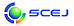English page## \$B9V1i?=9~\$N8!:w(B

\$B!&=jB0N,>N!W!"!V%-!<%o!<%I!W\$K\$D\$\$\$F8!:w\$G\$-\$^\$9!#(B

### \$B8!:w>r7o(B

\$B\$K(B \$B\$H\$\$\$&(B \$B\$r4^\$`(B
\$B\$?\$@\$7!"(B \$B7o\$^\$G\$7\$+I=<(\$7\$J\$\$(B

\$B%"%k%U%!%Y%C%H\$NBgJ8;z\$H>.J8;z\$O6hJL\$5\$l\$^\$;\$s!#(B
Perl \$B\$N@55,I=8=\$rMQ\$\$\$?8!:w\$b2DG=\$G\$9!#(B Perl \$B\$N@55,I=8=\$G\$O!"(B . ^ \$ \ * + ? [ ] ( ) | \$B\$N3FJ8;z\$K\$O(B \$BFCl9g\$O(B \$B\$=\$NJ8;z\$NA0\$K(B \ \$B\$rIU\$1\$F\$/\$@\$5\$\$!#(B

\$B2=3X9)3X2q(B \$BBh(B48\$B2s=)5(Bg2q(B (\$BFAEg(B 2016)(C) 2016 \$B8x1W
Most recent update: 2016-05-07 08:50:16
This page was generated byeasp 2.39; mknote 2.39 (C)1999-2016 kawase